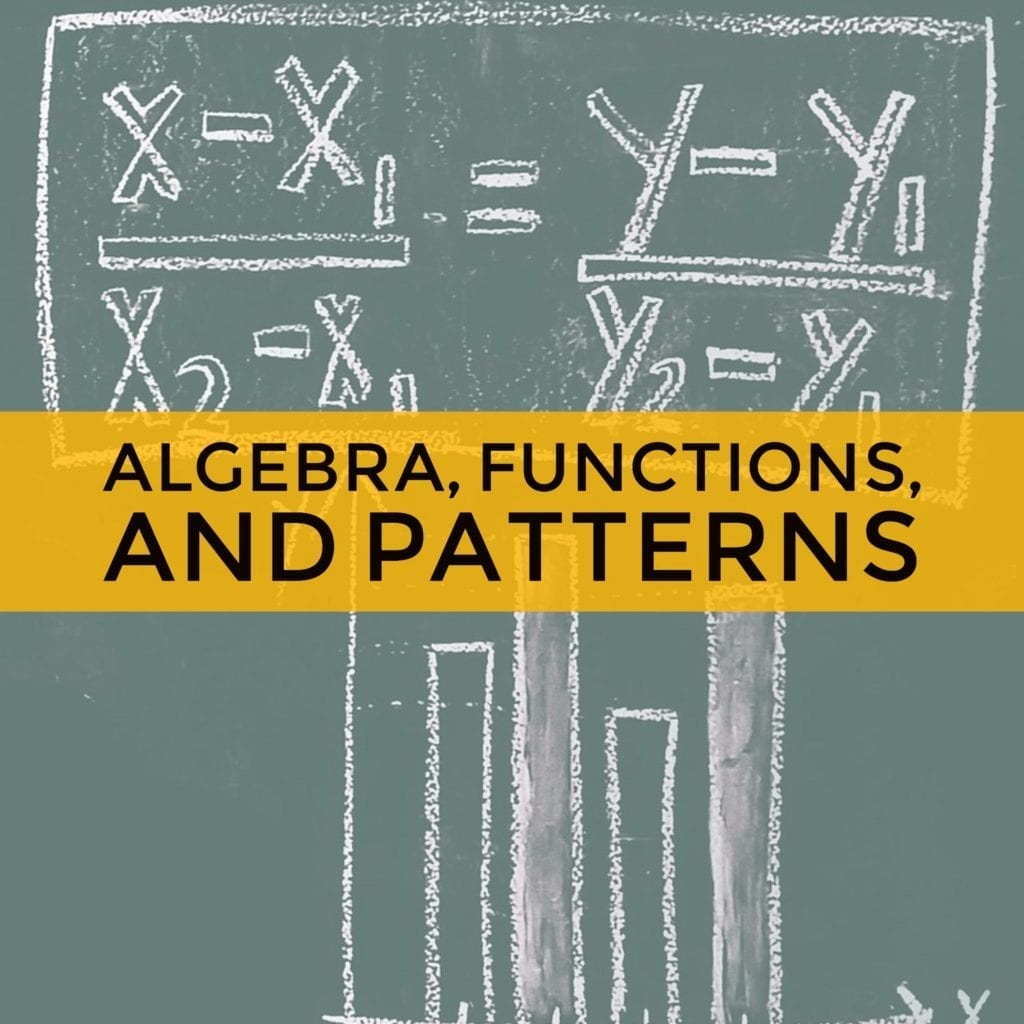# Algebra, Functions, and Patterns

Algebra, functions, & patterns cover 20-30% of the GED Math test.

Algebra

Algebra is a branch of mathematics that uses symbols to represent numbers and values in equations. These symbols represent variables, or quantities that don’t have fixed values. In algebra, when there is an unknown number in an equation, it is often represented by any letter of the alphabet. The most common letter you’ll see is ”˜x’.

A basic algebraic equation would look like this:

12 + 15 = x

The ”˜x‘ here represents the unknown value. The goal of algebra is to solve the equation in order to find the unknown value.

Function

An algebraic function is a type of equation that involves mathematical operations such as addition, subtraction, multiplication, division, and rational exponents. A function is a relation that connects one set to another set. A set simply is a collection of things e.g., a set of odd numbers {1, 3, 5, 7}.

There are many types of algebraic functions, namely:

1. Linear Function – a function defined by this equation: y = mx + c.
2. Quadratic Function – a function defined by this equation: f(x) = ax2 + bx + c, a â‰  0.
3. Polynomial – the sum or difference of monomials: f (x) = a n x n + a n-1 x n-1 + … + a 2 x 2 + a 1 x + a 0 , where a n, a n-1,…, a 1, a 0 are real numbers, n is a nonnegative integer, and a nâ‰  0 .
4. Cubic Function – a  function defined by a polynomial of degree 3. This is the form of cubic function:

f(x) = ax3 + bx2 + cx + d, a â‰  0.

1. Rational Function – a function that can be expressed as the quotient of two polynomial functions, written in the form of a/b.

Pattern

A pattern in mathematics is an arrangement of numbers and terms that are a result of following a specific rule. The sequence of numbers or terms are analyzed and studied to determine the rule that was used to create the pattern.

Here are some examples of algebra problems that you might encounter in the GED test:

1. Let f(x) = 2x 2 – 4x + 4 and h(x) = 2x – 4. Find:

a) f(4) =

b) h(3) =

c) f(3) + h(3) =

a) f(4) = 2(4) 2 – 4(4) + 4 = 12

b) h(3) = 2(3) – 4 = 2

c) f(3) + h(3) = 2(3) 2 – 4(3) + 4 + 2(3) – 4 = 12

1. 2x + 4 (-4) = -10

Solution:

2x – 16 = – 10

2x = 6

x = 3
For more tips and lessons on Algebra, Functions, and Patterns, watch our GED Math video lessons and take our GED Math practice test. Our practice tests include the answers to all math problems.